# Exponential equations online calculatorExponential is called an equation where unknown variable is in power, for example: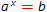To solve such equations different approaches are used, one of which is taking the logarithm. For instance, take the logarithm from both sides of the equation above.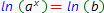According to the logarithm features: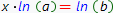Find: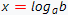The example above is the simplest one. Our online calculator, build on Wolfram Alpha system is able to solve almost any exponential equations with step by step solution.

Exponential equations solver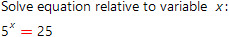Exponential equation you want to solve: